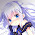$$\{0,1,2,3,4,5,6,7,8,9,…\}$$

 編號 菜籃 { $0$, $1$, $2$, $3$, $4$, $5$, $6$, $7$, $8$, $9$, ... } $0$ o y o y o o o o o o ... $1$ y o y o o o o o o o ... $2$ y y o o y y o o o o ... $3$ y o y y y o o o o o ... $4$ o o y y o y o o o o ... $5$ y y y o y y o o o o ... ⋮ ⋮

 編號 菜籃 { $0$, $1$, $2$, $3$, $4$, $5$, $6$, $7$, $8$, $9$, ... } $0$ o y o y o o o o o o ... $1$ y o y o o o o o o o ... $2$ y y o o y y o o o o ... $3$ y o y y y o o o o o ... $4$ o o y y o y o o o o ... $5$ y y y o y y o o o o ... ⋮ ⋮

 編號 菜籃 { $0$, $1$, $2$, $3$, $4$, $5$, ... } $?$ o o o y o y ...

 編號 菜籃 { $0$, $1$, $2$, $3$, $4$, $5$, ... } $?$ y y y o y o ...

#### 2 則留言:

1.“把所有自然數，從 0 開始，收集起來，放在一個菜籃裡，用大括號表示菜籃的範圍”
從一開始就是鬼扯，無窮集合根本是亂扯。
連No0 的 oyoyooooo... 菜籃根本就沒有從來成功被建構過
證明當然是鬼扯，不過相信Cantor那套鬼扯就相信吧，數學已經離經叛道了...

1.你的"經"以及"道"所謂何者？
數學本就是存於純粹理性中的東西
你可以不接受、不理解
但別把自己不接受的東西說成鬼扯
那只顯得自己更無知